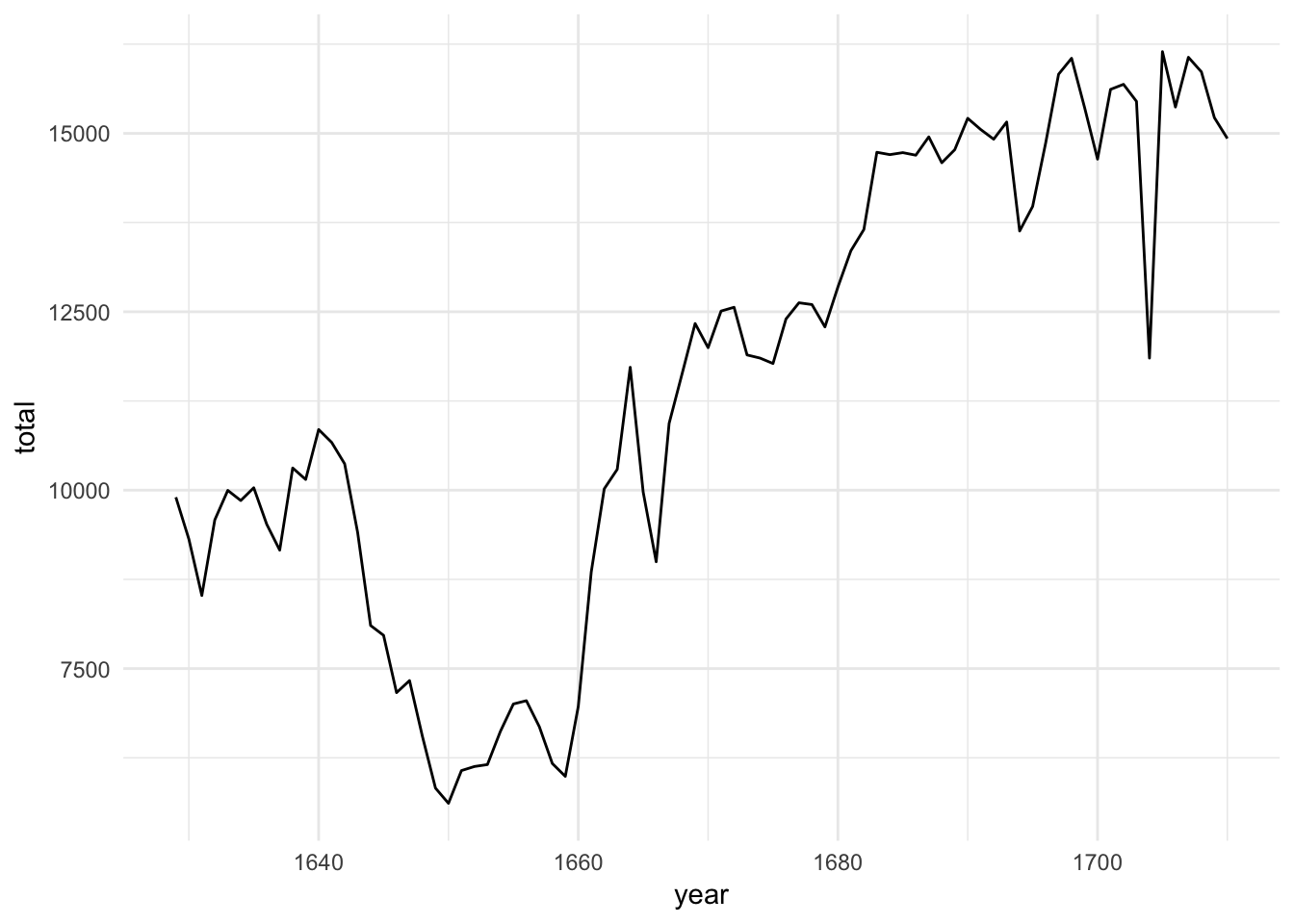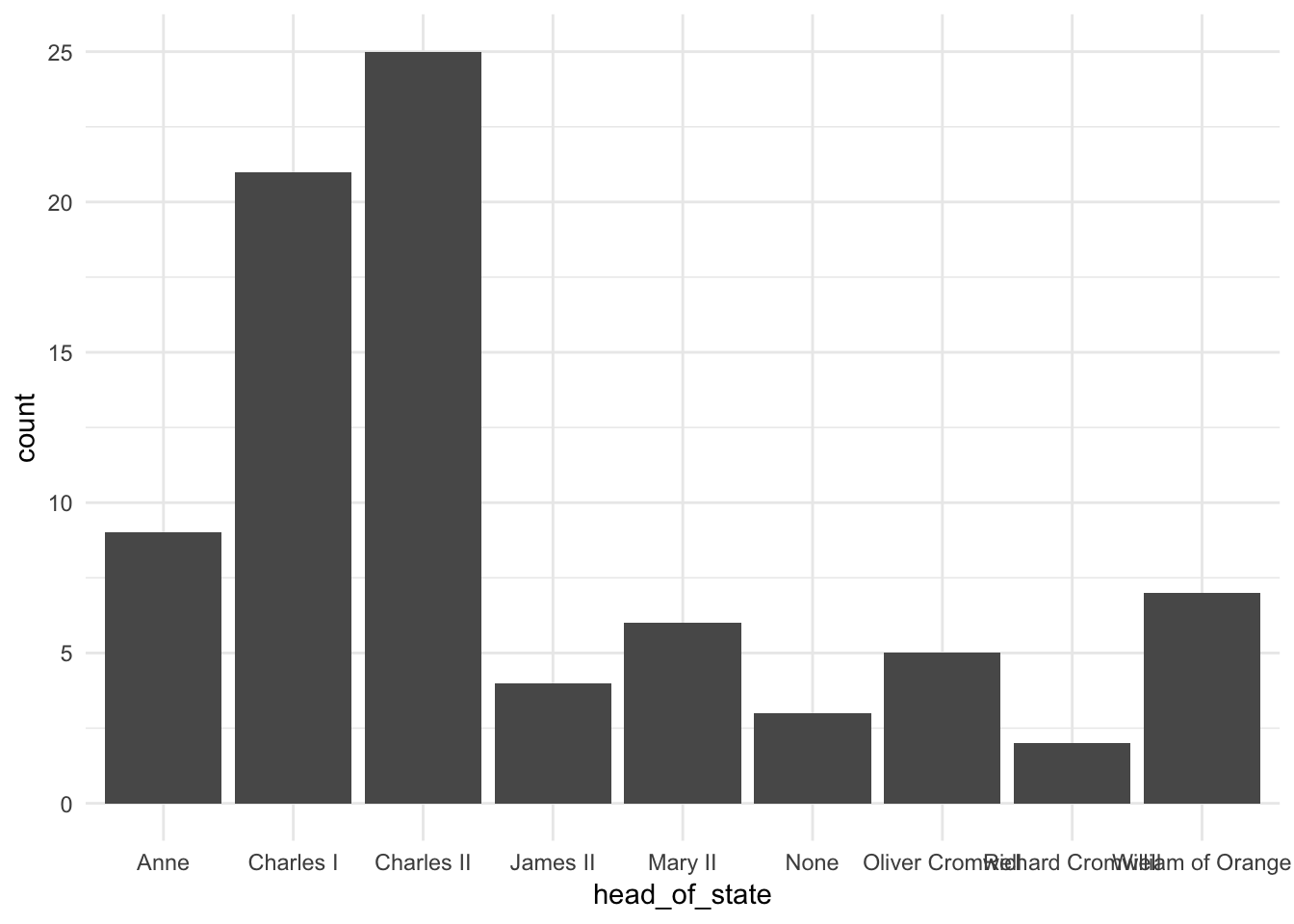The lab for today has you look at an exploratory analysis in R from two datasets. It introduces a lot of commands for data visualization that we will cover in detail next time.

### Import and view the data

The dataset here shows the number of children born in London on an annual basis, separated by their sex. Interactively in RStudio, you can see the data as a table by clicking on the table in the upper right-hand corner.

``births <- read_csv("https://statsmaths.github.io/stat_data/arbuthnot.csv")``

What years are included in the study?

Answer: We have data from 1629 to 1710.

Where/how do you think this data may have been collected?

Answer: It was actually collected through church baptism records, by far the most complete record of births in England at the time.

### Plots

Now, let’s visualize the dataset by constructing several different plots. We will learn later how the plotting mechanism actually works. Today just run the code and enjoy.

``````ggplot(births, aes(year, total)) +
geom_line()``````Approximately how many children were born in London in 1701?

Answer: 15 thousand

What is one change you would like to make to the way R has constructed the plot?

Answer: Add better labs for the x and y axes.

Click on the data in the top right hand pane. This will open an Excel like view of the dataset. Describe what the variable `head_of_state` most likely means:

Answer: It means who was the head of state of the government in control of London. This changed, rather drastically, throughout the timeperiod of the dataset.

### Variables

You might also have noticed the row of three letter abbreviations under the column names. These describe the type of each variable:

• `int` stands for integers.
• `dbl` stands for doubles, or real numbers.
• `chr` stands for character vectors, or strings.

The types of graphics and data manipulation we can do with a given variable is highly determined by the variable data types.

Explain in words why the variables `boys` and `girls` are interpreted as integers.

Answer: These variables are counts and it does not make sense for us to have non-integer numbers here (say, half a person).

### Bar Plot

Create a bar plot of the heads of state:

``````ggplot(births, aes(head_of_state)) +
geom_bar()``````Who was the longest serving head of state during the time period of this data set?

Answer: Charles II

How are the heads of state arranged be default?

Answer: Alphabetically.

### Color

We can add color to the line plot to combine the head of state information with the year and total number of births:

``````ggplot(births, aes(year, total)) +
geom_line(aes(color = head_of_state))``````